# Tutorial 7: Transient Flow Analysis using Feed Forward Controller Model

Purpose/Objective:

This exercise will show the user how to create a Feed Forward Controller that will adjust a valve opening position based on the valve upstream pressure. The user will learn how to:

• Create a Feed Forward Controller
• Setup a transient run
• Check the model
• Run the model
• Post-process the model
• Elements used: Conventional Orifice, Standard Tube, Ball Valve, FFWD Controller, Generic Fixed Volume Accumulator.

Step 1: Create plan

• The goal of this exercise is to determine time it takes a volume to pressurize up to 400 psi from a small leak and then how long it takes to depressurize the volume after a valve is opened by a feed forward controller.
1. Volume initial pressure = 14.7 psi
2. Fluid = air; Use compressible gas elements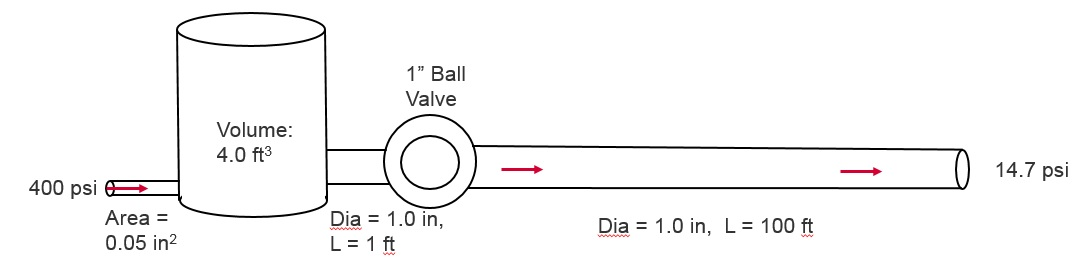Figure 1.01: Model Plan

Step 2: Building Model

1. Create Chambers (2 Boundary Plenum and 2 Internal Momentum) and the Accumulator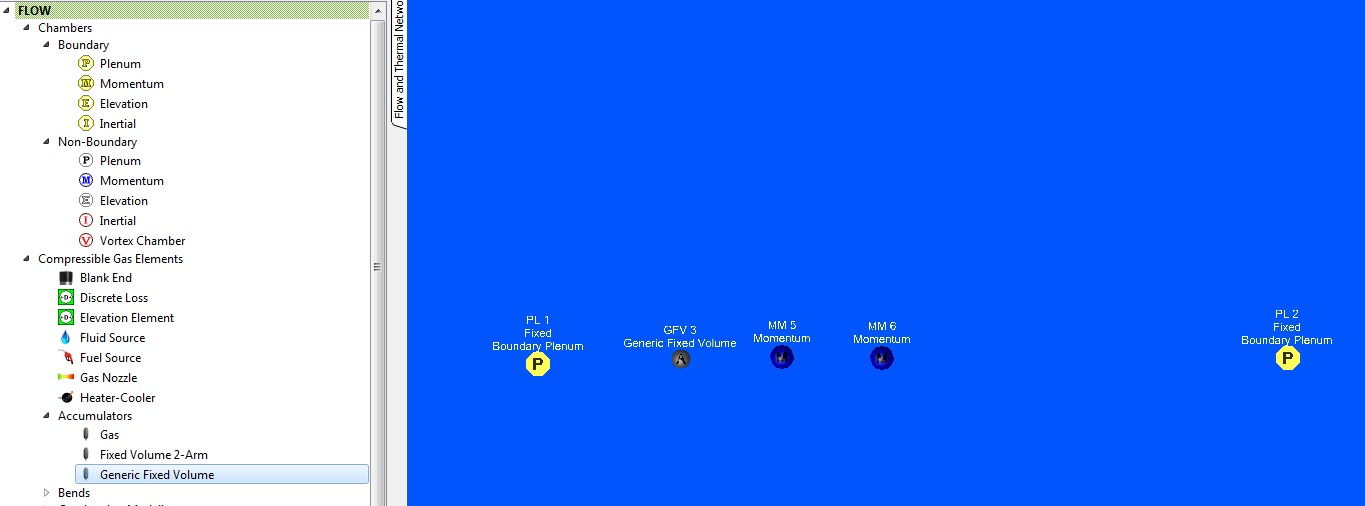Figure 1.02: Chamber and Accumulator

2. Create Elements: 1 Conventional Orifice, 2 Standard Tubes, and 1 Ball ValveFigure 1.03: Elements

3. Create Feed Forward Controller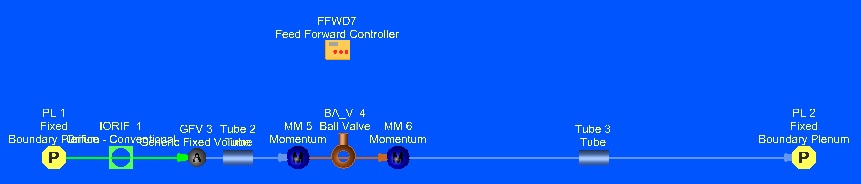Figure 1.04: Feed Forward Controller

4. Assign Chamber Pressure and Temperature
1. Upstream Boundary Plenum: P= 400 psi, T= 80 F
2. Downstream Boundary Plenum: P= 14.7 psi, T= 80 F
3. 2 Internal Momentum: Ptotal = 14.7 psi and Pstatic = 14.7 psi, T=80F.

These are the initial conditions for the transient.

5. Assign Accumulator Properties: Total Volume = 4 ft3
• Chamber Initialization for the accumulator: Plenum, Pstatic = 14.7 psi, T = 80F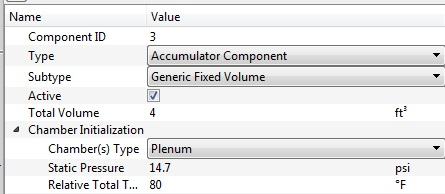Figure 1.05: Accumulator Properties

6. Assign Element properties
1. Orifice: Area = 0.05 in2
2. Upstream Tube: Dia = 1 in., Length = 1 ft, Heat Transfer = Adiabatic
3. Downstream Tube: Dia = 1 in, Length = 100 ft, Heat Transfer = Adiabatic
4. Ball Valve: Pipe Dia = 1 in, Valve Position = 0% open (controller will adjust this)
7. Assign Feed Forward Controller Properties
1. Controller will use a python script.

# Valve position will be set to 100% open if the upstream pressure> 400 psi.

# After the valve position is 100% open it will stay 100% open until the run ends.

if VALVEPOS_CUR == 0.0:

if PS > 400:

VALVEPOS_NEW=100

else:

VALVEPOS_NEW=0

else:

VALVEPOS_NEW=100

8. Copy and Paste or retype the text. Lines with # are comments. Python uses indentation to identify control logic (if and else statements).
9. 2-gauge variables: Upstream pressure and the ball valve position
• Ball valve position will be used as a gauge and manipulated variable.
10. 1 manipulated variable: the ball valve position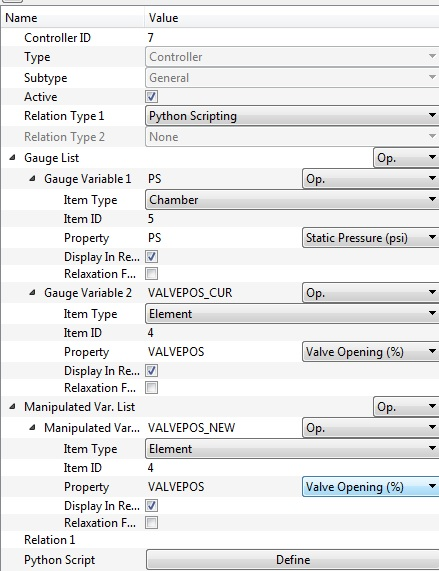Figure 1.06: FFWD Controller properties

11. Analysis Setup:
1. Analysis Type: Transient Flow
2. Initial Condition Option: User-defined Pressure and Temperature Data
• Solver will use P and T that have been assigned to the 2 internal momentum chambers and the accumulator for the time = 0 sec. solution.
3. Transient Run Control Parameters (Figure 1.07)
1. Run Start Time: 0 sec
2. Run Stop Time: 100 sec
3. Solution Time Step Option: Automatic Time Stepping
• Initial, Minimum and Maximum Time Step: 0.01, 0.1, 0.5 sec
4. Output Time Step Option: Constant Output Intervals
• Output Time Step: 0.5 sec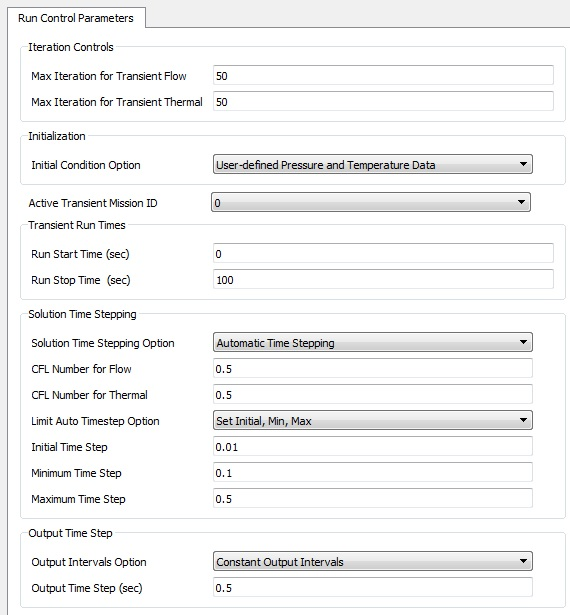Figure 1.07: Transient Run Control Parameters

Step 3: Check Model and Run

1. Select checkmark icon from the top toolbar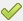to check the model for warnings/errors.
• No errors or warnings should appear.
2. Select run icon from toolbar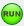. Run Flow Simulator.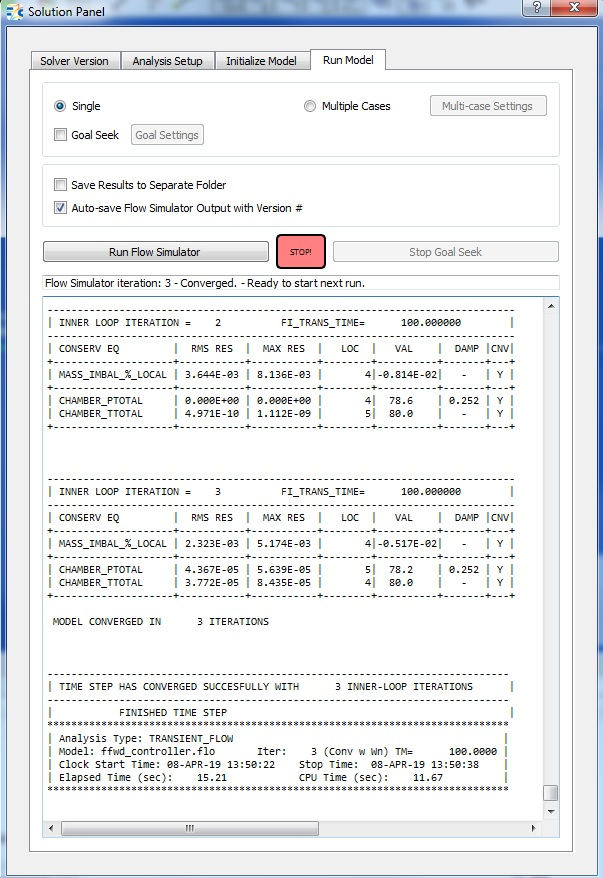Figure 1.08: Run Panel

Step 4: Post-process

1. The first results file (*.res) of the transient should automatically be loaded into GUI. If not, it can be selected via File → Load Result File
2. By default, both chamber and elemental results are displayed in the graphical workspace.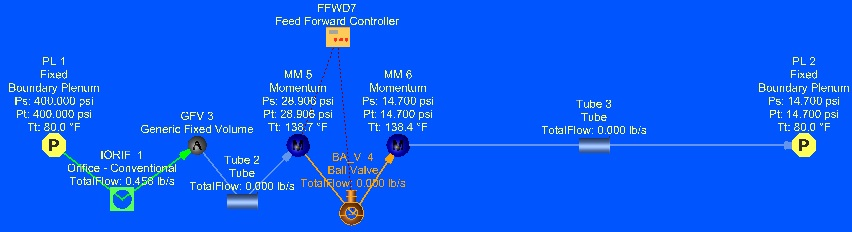Figure 1.09: Results at 0.5 seconds

3. Use the Results Table for post processing results Versus Time.
1. Pick the Accumulator Static Pressure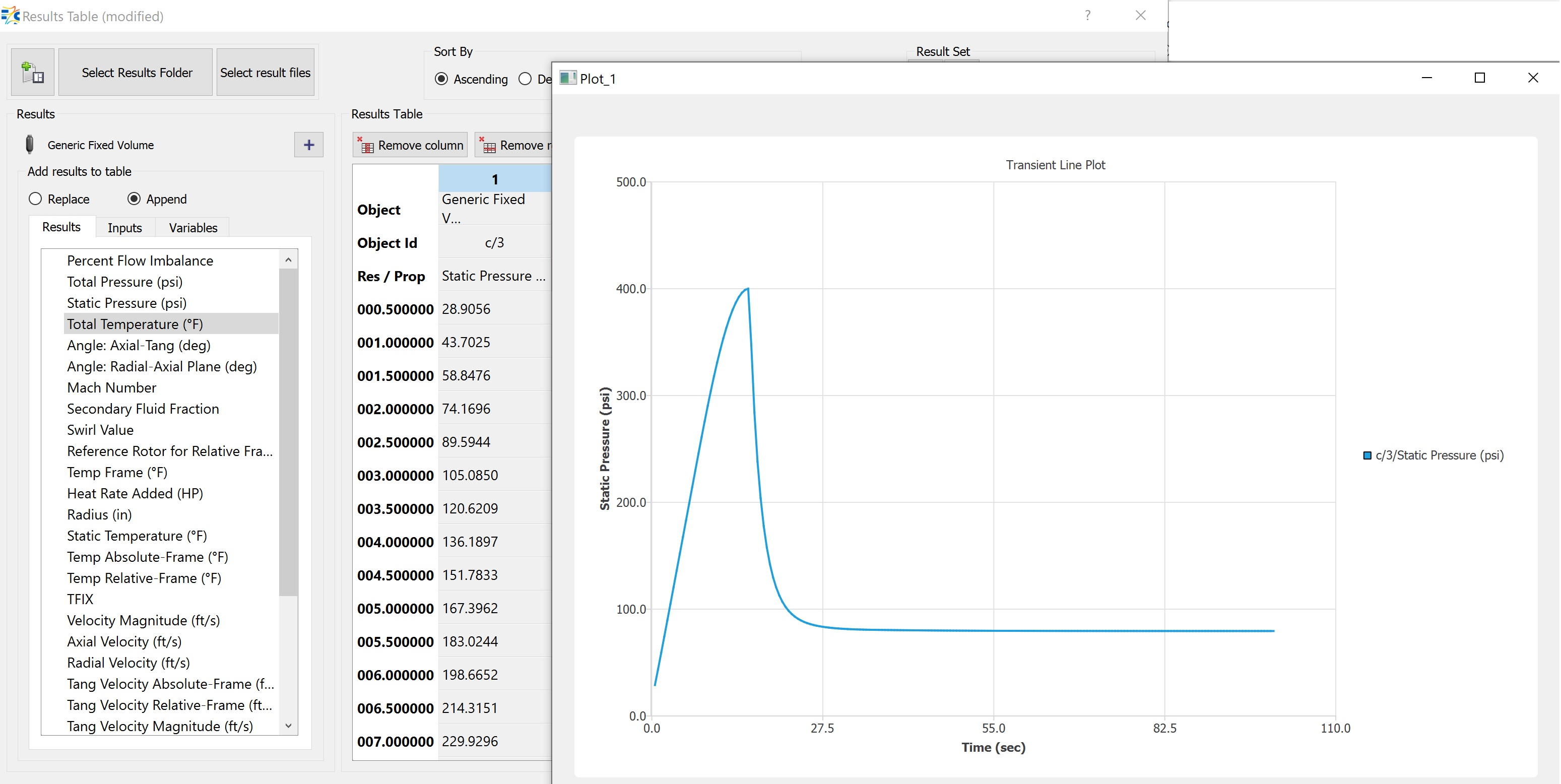Figure 1.10: Accumulator Static Pressure versus Time

2. Pick the Orifice element upstream of the accumulator and the tube downstream and plot Total Flow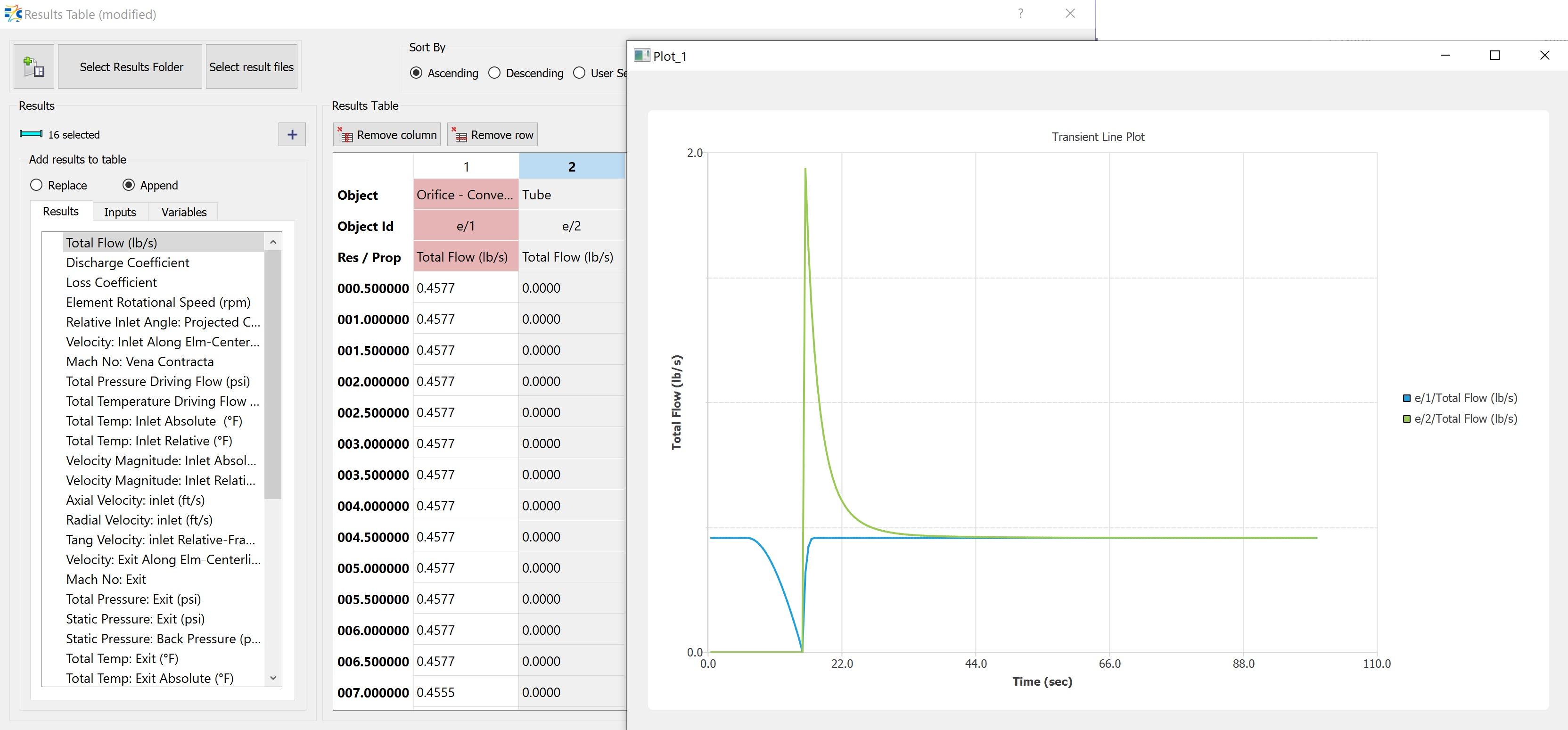Figure 1.11: